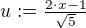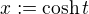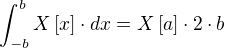## Partial integration

To differentiate a product:By integrating this:## ForTo get the idea, compare differentiation:.

## ForTo calculate, complete the square and substitute, for example:Substitute.The goal is to rewrite the integral by substitution in order for it to take one of these forms:

•••## ForTo calculate, complete the square and substitute (using a linear substitution) in order for it to take one of these forms:

••••## For## Forwith.Calculate J by substituting.The equationhas no real solutions.

Calculate K by substituting to arrive at:If, then:For, recurse.

Let R(x) be a rational function.

## ForSubstituteto get a rational function in t.

## ForSubstituteto get a rational function in t.

## ForSubstituteto get a rational function in t.

## ForSubstituteto get an exponential integral.

## ForSubstituteto get an exponential integral.

## ForSubstituteorto get a function with just sin and cos in them.

## Forcomplete the square.

## Forwith a polynom P

partial integration to decrease the order of the polynom.

## Forwith a polynom P

partial integration to decrease the order of the polynom.

## Forwith a polynom P

partial integration to decrease the order of the polynom.

## Uneigentliche Integrale

### Locally Integrable

A function is locally integrable if it is at least integrable in a range [a,x).

One can also do this with the range (x,a].

### Limit

By crossing the border, one can define the Uneigentliches Integral like this:## Multi-dimensional partial integration

Let Ω be a subset of R^n with a piecemeal smooth border ∂Ω. Let the orientation of the border be the normal vector n.

Let v be a differentiable vector field on the environment Ω and φ a steady differentiable scalar field on Ω.

Let the abbreviation dS mean n⋅dS.

Then follows, reminiscent of the product integral rule:## Estimating Integrals## Connection between Integrals and Differentialswhere.